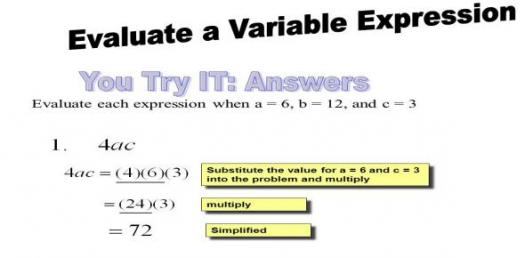# Variable And Expression Trivia Quiz

10 Questions | Attempts: 180SettingsIn mathematics, a variable is a symbol with a value. It is regarded as a variable because it values can change. Mathematical expressions contain one or more variables. It is different from mathematical equations because the value of all variables is not equated. Are you sound in mathematical expressions? Let's take a quiz!Back to top